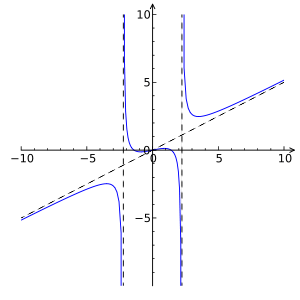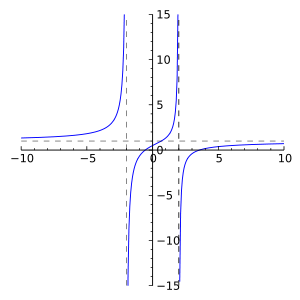# Rational function

For the use in automata theory, see Finite-state transducer. For the use in monoid theory, see Rational function (monoid).

In mathematics, a rational function is any function which can be defined by a rational fraction, i.e. an algebraic fraction such that both the numerator and the denominator are polynomials. The coefficients of the polynomials need not be rational numbers, they may be taken in any field K. In this case, one speaks of a rational function and a rational fraction over K. The values of the variables may be taken in any field L containing K. Then the domain of the function is the set of the values of the variables for which the denominator is not zero and the codomain is L.

The set of rational functions over a field K is a field, the field of fractions of the ring of the polynomial functions over K.

## Definitions

A functionis called a rational function if and only if it can be written in the formwhereandare polynomials inandis not the zero polynomial. The domain ofis the set of all pointsfor which the denominatoris not zero.

However, ifandhave a non constant polynomial greatest common divisor, then settingandproduces a rational functionwhich may have a larger domain than, and is equal toon the domain ofIt is a common usage to identifyand, that is to extend "by continuity" the domain ofto that ofIndeed, one can define a rational fraction as an equivalence class of fractions of polynomials, where two fractions A(x)/B(x) and C(x)/D(x) are considered equivalent if A(x)D(x) = B(x)C(x). In this caseis equivalent to.

## Examples

Examples of rational functionsRational function of degree 3:Rational function of degree 2:The rational functionis not defined at. It is asymptotic toas x approaches infinity.

The rational functionis defined for all real numbers, but not for all complex numbers, since if x were a square root of(i.e. the imaginary unit or its negative), then formal evaluation would lead to division by zero:, which is undefined.

A constant function such as f(x) = π is a rational function since constants are polynomials. Note that the function itself is rational, even though the value of f(x) is irrational for all x.

Every polynomial functionis a rational function with. A function that cannot be written in this form, such as, is not a rational function. The adjective "irrational" is not generally used for functions.

The rational functionis equal to 1 for all x except 0, where there is a removable singularity. The sum, product, or quotient (excepting division by the zero polynomial) of two rational functions is itself a rational function. However, the process of reduction to standard form may inadvertently result in the removal of such singularities unless care is taken. Using the definition of rational functions as equivalence classes gets around this, since x/x is equivalent to 1/1.

## Taylor series

The coefficients of a Taylor series of any rational function satisfy a linear recurrence relation, which can be found by setting the rational function equal to its Taylor series and collecting like terms.

For example,Multiplying through by the denominator and distributing,After adjusting the indices of the sums to get the same powers of x, we getCombining like terms givesSince this holds true for all x in the radius of convergence of the original Taylor series, we can compute as follows. Since the constant term on the left must equal the constant term on the right it follows thatThen, since there are no powers of x on the left, all of the coefficients on the right must be zero, from which it follows thatConversely, any sequence that satisfies a linear recurrence determines a rational function when used as the coefficients of a Taylor series. This is useful in solving such recurrences, since by using partial fraction decomposition we can write any rational function as a sum of factors of the form 1 / (ax + b) and expand these as geometric series, giving an explicit formula for the Taylor coefficients; this is the method of generating functions.

## Abstract algebra and geometric notion

In abstract algebra the concept of a polynomial is extended to include formal expressions in which the coefficients of the polynomial can be taken from any field. In this setting given a field F and some indeterminate X, a rational expression is any element of the field of fractions of the polynomial ring F[X]. Any rational expression can be written as the quotient of two polynomials P/Q with Q ≠ 0, although this representation isn't unique. P/Q is equivalent to R/S, for polynomials P, Q, R, and S, when PS = QR. However since F[X] is a unique factorization domain, there is a unique representation for any rational expression P/Q with P and Q polynomials of lowest degree and Q chosen to be monic. This is similar to how a fraction of integers can always be written uniquely in lowest terms by canceling out common factors.

The field of rational expressions is denoted F(X). This field is said to be generated (as a field) over F by (a transcendental element) X, because F(X) does not contain any proper subfield containing both F and the element X.

### Complex rational functions

In complex analysis, a rational functionis the ratio of two polynomials with complex coefficients, where Q is not the zero polynomial and P and Q have no common factor (this avoids f taking the indeterminate value 0/0). The domain and range of f are usually taken to be the Riemann sphere, which avoids any need for special treatment at the poles of the function (where Q(z) is 0).

The degree of a rational function is the maximum of the degrees of its constituent polynomials P and Q. If the degree of f is d, then the equationhas d distinct solutions in z except for certain values of w, called critical values, where two or more solutions coincide. The function f can therefore be thought of as a d-fold covering of the w-sphere by the z-sphere.

Rational functions with degree 1 are called Möbius transformations and form the automorphisms group of the Riemann sphere. Rational functions are representative examples of meromorphic functions.

### Notion of a rational function on an algebraic variety

Like polynomials, rational expressions can also be generalized to n indeterminates X1,..., Xn, by taking the field of fractions of F[X1,..., Xn], which is denoted by F(X1,..., Xn).

An extended version of the abstract idea of rational function is used in algebraic geometry. There the function field of an algebraic variety V is formed as the field of fractions of the coordinate ring of V (more accurately said, of a Zariski-dense affine open set in V). Its elements f are considered as regular functions in the sense of algebraic geometry on non-empty open sets U, and also may be seen as morphisms to the projective line.

## Applications

These objects are first encountered in school algebra. In more advanced mathematics they play an important role in ring theory, especially in the construction of field extensions. They also provide an example of a nonarchimedean field (see Archimedean property).

Rational functions are used in numerical analysis for interpolation and approximation of functions, for example the Padé approximations introduced by Henri Padé. Approximations in terms of rational functions are well suited for computer algebra systems and other numerical software. Like polynomials, they can be evaluated straightforwardly, and at the same time they express more diverse behavior than polynomials.

Rational functions are used to approximate or model more complex equations in science and engineering including (i) fields and forces in physics, (ii) spectroscopy in analytical chemistry, (iii) enzyme kinetics in biochemistry, (iv) electronic circuitry, (v) aerodynamics, (vi) medicine concentrations in vivo, (vii) wave functions for atoms and molecules, (viii) optics and photography to improve image resolution, and (ix) acoustics and sound.